Courses

# RD Sharma Solutions - Chapter 1 - Rational Numbers (Ex -1. 5), Class 8, Maths Class 8 Notes | EduRev

## RD Sharma Solutions for Class 8 Mathematics

Created by: Abhishek Kapoor

## Class 8 : RD Sharma Solutions - Chapter 1 - Rational Numbers (Ex -1. 5), Class 8, Maths Class 8 Notes | EduRev

The document RD Sharma Solutions - Chapter 1 - Rational Numbers (Ex -1. 5), Class 8, Maths Class 8 Notes | EduRev is a part of the Class 8 Course RD Sharma Solutions for Class 8 Mathematics.
All you need of Class 8 at this link: Class 8

Question 1: Multiply: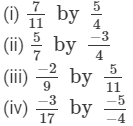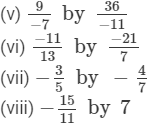(i)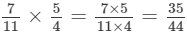(ii)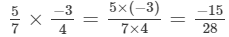(iii)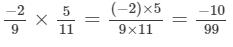(iv)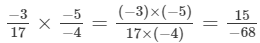(v)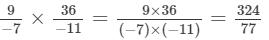(vi)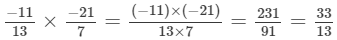(vii)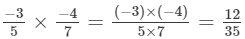(viii)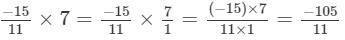Question 2: Multiply: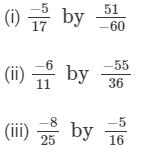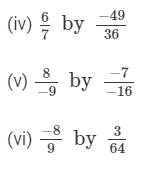(i)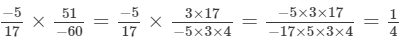(ii)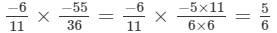(iii)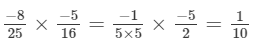(iv)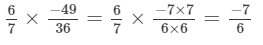(v)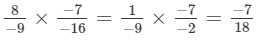(vi)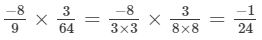Question 3: Simplify each of the following and express the result as a rational number in standard form: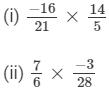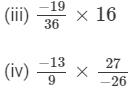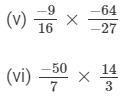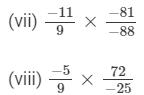(i)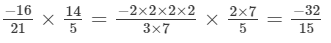(ii)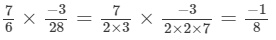(iii)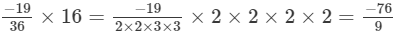(iv)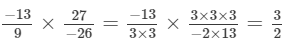(v)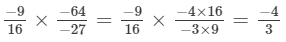(vi)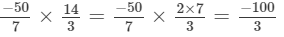(vii)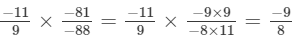(viii)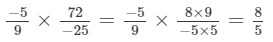Question 4: Simplify: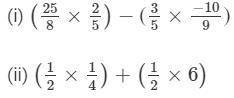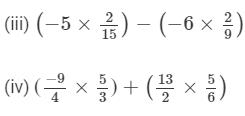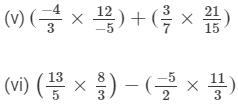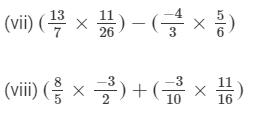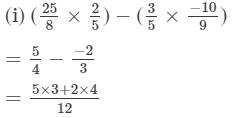= 23/12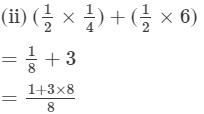= 25/8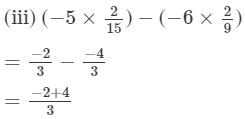= 2/3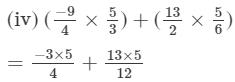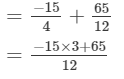= 20/12 = 5/3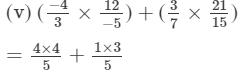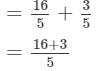= 19/5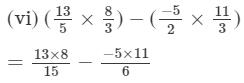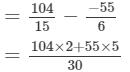= 483/30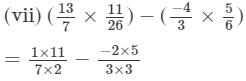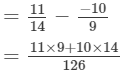= 239/126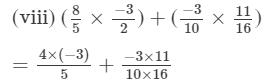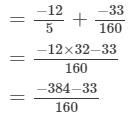= -417/160

Question 5: Simplify: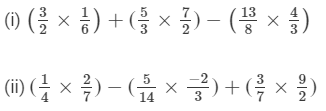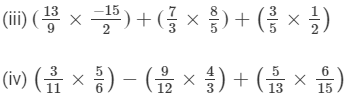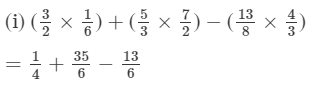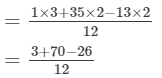= 47/12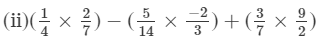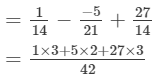= 94/42 = 47/21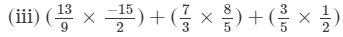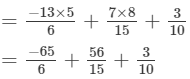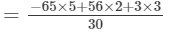= -204/30 = -34/5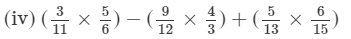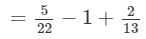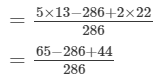= -177/ 286

91 docs

,

,

,

,

,

,

,

,

,

,

,

,

,

,

,

,

,

,

,

,

,

,

,

,

,

,

,

;